Home
Hostname: page-component-55597f9d44-5zjcf Total loading time: 0.241 Render date: 2022-08-18T17:33:03.784Z Has data issue: true Feature Flags: { "shouldUseShareProductTool": true, "shouldUseHypothesis": true, "isUnsiloEnabled": true, "useRatesEcommerce": false, "useNewApi": true } hasContentIssue true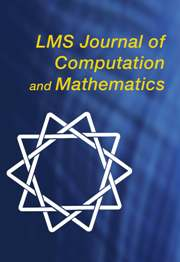LMS Journal of Computation and Mathematics

# Rational functions with maximal radius of absolute monotonicity

Published online by Cambridge University Press:  01 May 2014

## Abstract

HTML view is not available for this content. However, as you have access to this content, a full PDF is available via the ‘Save PDF’ action button.

We study the radius of absolute monotonicity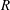$R$ of rational functions with numerator and denominator of degree$s$ that approximate the exponential function to order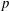$p$. Such functions arise in the application of implicit$s$-stage, order$p$ Runge–Kutta methods for initial value problems, and the radius of absolute monotonicity governs the numerical preservation of properties like positivity and maximum-norm contractivity. We construct a function with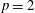$p=2$ and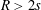$R>2s$, disproving a conjecture of van de Griend and Kraaijevanger. We determine the maximum attainable radius for functions in several one-parameter families of rational functions. Moreover, we prove earlier conjectured optimal radii in some families with two or three parameters via uniqueness arguments for systems of polynomial inequalities. Our results also prove the optimality of some strong stability preserving implicit and singly diagonally implicit Runge–Kutta methods. Whereas previous results in this area were primarily numerical, we give all constants as exact algebraic numbers.

Type
Research Article
Information

## References

Bolley, C. and Crouzeix, M., ‘Conservation de la positivité lors de la discrétisation des problémes d’évolution paraboliques’, RAIRO Anal. Numér. 12 (1978) 237245.CrossRefGoogle Scholar
Butcher, J. C., Numerical methods for ordinary differential equations , 2nd edn (Wiley, 2008).CrossRefGoogle Scholar
Ferracina, L. and Spijker, M. N., ‘Strong stability of singly-diagonally-implicit Runge–Kutta methods’, Appl. Numer. Math. 58 (2008) 16751686.CrossRefGoogle Scholar
Gottlieb, S., Ketcheson, D. I. and Shu, C.-W., Strong stability preserving Runge–Kutta and multistep time discretizations (World Scientific, 2011).CrossRefGoogle Scholar
van de Griend, J. A. and Kraaijevanger, J. F. B. M., ‘Absolute monotonicity of rational functions occurring in the numerical solution of initial value problems’, Numer. Math. 49 (1986) 413424.CrossRefGoogle Scholar
Hairer, E. and Wanner, G., Solving ordinary differential equations II: stiff and differential-algebraic problems (Springer, 1991).CrossRefGoogle Scholar
Ketcheson, D. I., ‘Computation of optimal monotonicity preserving general linear methods’, Math. Comp. 78 (2009) 14971513.CrossRefGoogle Scholar
Ketcheson, D. I., ‘High order strong stability preserving time integrators and numerical wave propagation methods for hyperbolic PDEs’, PhD Thesis, University of Washington, 2009.Google Scholar
Ketcheson, D. I., Macdonald, C. B. and Gottlieb, S., ‘Optimal implicit strong stability preserving Runge–Kutta methods’, Appl. Numer. Math. 59 (2009) 373392.CrossRefGoogle Scholar
Kraaijevanger, J. F. B. M., ‘Absolute monotonicity of polynomials occurring in the numerical solution of initial value problems’, Numer. Math. 48 (1986) 303322.CrossRefGoogle Scholar
Kraaijevanger, J. F. B. M., ‘Contractivity of Runge–Kutta Methods’, BIT 31 (1991) 482528.CrossRefGoogle Scholar
Spijker, M. N., ‘Contractivity in the numerical solution of initial value problems’, Numer. Math. 42 (1983) 271290.CrossRefGoogle ScholarYou have Access
2
Cited by

# Save article to Kindle

Note you can select to save to either the @free.kindle.com or @kindle.com variations. ‘@free.kindle.com’ emails are free but can only be saved to your device when it is connected to wi-fi. ‘@kindle.com’ emails can be delivered even when you are not connected to wi-fi, but note that service fees apply.

Find out more about the Kindle Personal Document Service.

Rational functions with maximal radius of absolute monotonicity
Available formats
×

# Save article to Dropbox

To save this article to your Dropbox account, please select one or more formats and confirm that you agree to abide by our usage policies. If this is the first time you used this feature, you will be asked to authorise Cambridge Core to connect with your Dropbox account. Find out more about saving content to Dropbox.

Rational functions with maximal radius of absolute monotonicity
Available formats
×

# Save article to Google Drive

To save this article to your Google Drive account, please select one or more formats and confirm that you agree to abide by our usage policies. If this is the first time you used this feature, you will be asked to authorise Cambridge Core to connect with your Google Drive account. Find out more about saving content to Google Drive.

Rational functions with maximal radius of absolute monotonicity
Available formats
×
×